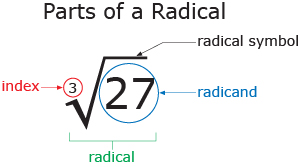# Math help from the Learning Centre

This guide provides useful resources for a wide variety of math topics. It is targeted at students enrolled in a math course or any other Centennial course that requires math knowledge and skills.

The term radical refers to an expression involving the $$\sqrt{}$$ symbol that is placed on numbers to represent the "square root" or "$$n$$th root of a number".

## Square Root

The square root of a number is the value that you can multiply by itself twice to obtain the original number.

Examples

$$\sqrt{4} = 2$$ because $$2 \times 2 = 4$$.
$$\sqrt{25} = 5$$ because $$5 \times 5 = 25$$.

## nth Root

The $$n$$th root of a number is the value that you can multiply by itself $$n$$ times to obtain the original number.

The index of a radical is what denotes the value of $$n$$. (So when $$n=2$$, we just have a square root.)Examples

$$\sqrt{27} = 3$$ because $$3 \times 3 \times 3 = 3^3 = 27$$.
$$\sqrt{16} = 2$$ because $$2 \times 2 \times 2 \times 2 = 2^4 = 16$$.
$$\sqrt{-64} = -4$$ because $$(-4) \times (-4) \times (-4) = (-4)^3 = -64$$.

$$\sqrt{-16}$$ produces a value that is not a real number. The reason why is because it is impossible to multiply the same number by itself and obtain a negative number. Recall that when two negatives multiply by each other we obtain a positive value.

Unless you are studying complex/imaginary numbers (see Complex Numbers), we just say that we cannot obtain a real value and recognize this as being unsolvable. So, you cannot take an $$n$$th root of a negative number if $$n$$ is even.

An expression is in radical form if it includes the radical symbol.
An expression is in power/exponent form if it includes exponents and NO radical symbol.

Radicals and exponents can be connected using the following important formula:

\begin{align}
\end{align}

If $$a$$ is negative, then there are certain limitations to this rule that are explained in the next subsection.

Notice how if $$m = 1$$ the above formula becomes:

\begin{align}
a^{\frac{1}{n}} &= \sqrt[n]{a}
\end{align}

Both (1) and (2) are valid. Choosing between which version of the formula to use depends on what expression you're working with. Sometimes it's easier to use (1) and sometimes it's easier to use (2). Choose whichever one YOU think will be easier to work with at the time. However, when writing down a final answer in radical form, use (1).

The left-hand-side is in exponent form, and the right-hand-side of (1) and (2) are in radical form.

Example 1

Evaluate $$\left(-4\right)^{\frac{4}{2}}$$.

Solution

\begin{align}          \left(-4\right)^{\frac{4}{2}} &= \left(-4\right)^2 \\ &= 16     \end{align}

As the problem is given to us in exponent form, we are not applying the power-of-a-power rule and are just simplifying the exponent to solve the question.

Example 2

Evaluate $$\left(\sqrt{-81}\right)^2$$.

Solution

We cannot take the square root of a negative number, so we cannot arrive at a solution if we are only working with real numbers.

We CANNOT use power-of-a-power rule in this scenario because that rule is not applicable to negative bases with even-index radicals (such as the square root).

\begin{align}          \left(\sqrt{-81}\right)^2 &\neq \left(-81\right)^\frac{2}{2}     \end{align}

If you are working with complex numbers, the answer does end up being $$-81$$ but it is NOT due to the use of power-of-a-power rule.

If you are curious, this is the solution using complex numbers:

\begin{align}          \left(\sqrt{-81}\right)^2 &= \left(9i\right)^2 \\ &= 81i^2 \\ &= -81     \end{align}

Example 3

Evaluate $$\left(\sqrt{-27}\right)^2$$.

Solution

\begin{align}        \left(\sqrt{-27}\right)^2 &= \left(-3\right)^2 \\ &= 9      \end{align}

In this scenario, it would have been okay to use the power-of-a-power rule because the negative base (-27) had a radical with an odd index (3). However, we did not need to because it is easier to evaluate this problem like we did above then to try and evaluate $$\left(-27\right)^\frac{2}{3}$$

Example 4

Evaluate $$\left(\sqrt{-3}\right)^{36}$$.

Solution

\begin{align}        \left(\sqrt{-3}\right)^{36} &= \left(-3\right)^\frac{36}{9} \\ &= \left(-3\right)^4 \\ &= 81      \end{align}

It is okay to use the power-of-a-power rule here because the negative base (-3) had a radical with an odd index (9).

Example 1

Rewrite $$\sqrt{3}$$ in exponent form.

Solution

\begin{align} \sqrt{3} &= 3^{\frac{1}{2}} \end{align}

Remember that a square root has an index of 2.
We don't write a little "two" because the square root is universally recognized as being a radical with index two.

Example 2

Rewrite $$\sqrt{17}$$ in exponent form.

Solution

\begin{align} \sqrt{17} &= 17^{\frac{1}{3}} \end{align}

This radical has an index of 3.

Example 3

Rewrite $$\sqrt{x^3}$$ in exponent form.

Solution

\begin{align} \sqrt{x^3} &= x^{\frac{3}{2}} \end{align}

Our solution is also the answer to:
Rewrite $$\left(\sqrt{x}\right)^3$$ in exponent form.

Example 4

Rewrite $$x^{\frac{5}{7}}$$ in radical form.

Solution

\begin{align} x^{\frac{5}{7}} &= \sqrt{x^5} \end{align}

While $$\left(\sqrt{x}\right)^5$$ is equivalent to our solution, it is preferred to have our solution be written as it is above.

Radicals can be added and subtracted in a very similar way to how we add/subtract regular algebraic expressions.

Example

$$3\sqrt{2}$$ , $$11\sqrt{2}$$ , and $$-4\sqrt{2}$$ , are all like radicals.

$$3\sqrt{2} + 11\sqrt{2} - 4\sqrt{2} = 10\sqrt{2}$$

Example

Between, $$3\sqrt{2}$$ , $$11\sqrt{3}$$ , and $$-4\sqrt{2}$$ , none are like radicals.

$$3\sqrt{2} + 11\sqrt{3} - 4\sqrt{2}$$ cannot be simplified further.

To know how to express radicals such as $$\sqrt{54}$$ or $$5\sqrt{180}$$ in lowest terms using the rules we've laid out so far, see the next section on Reducing Radicals.